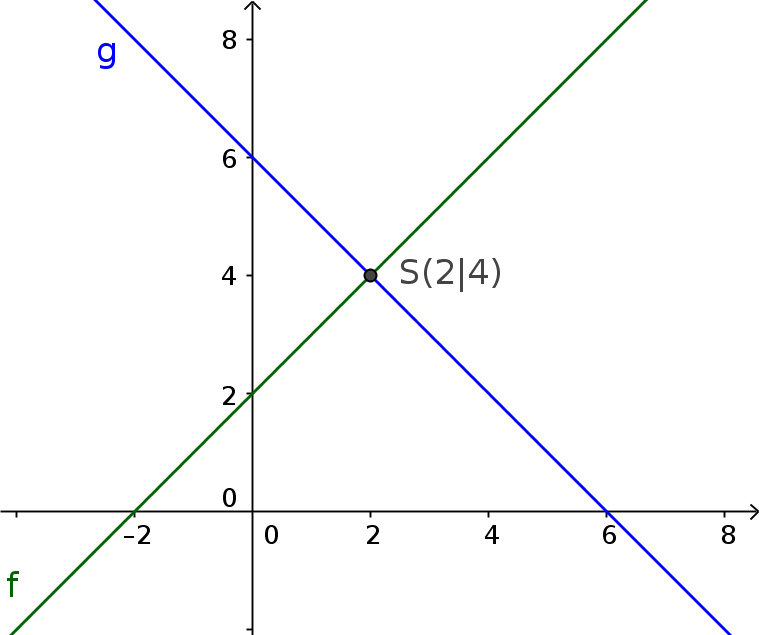Math Systems of linear equations Graphical method

# Graphical method

In the graphical method one imagines the linear equations as a linear function.

!

### Remember

A system of linear equations can have different solutions, which can be found graphically as follows:
• one solution: the straight lines intersect at one point
• no solution: the straight lines are parallel to each other
• infinitely many solution: the straight lines are identical
i

### Method

1. Convert the equations appropriately.
2. Draw the graphs of the equations into a coordinate system.

### Example

Determine graphically the solution set of the system of linear equations:

1. $4x=4y-8$
2. $y-6=-x$
1. #### Convert the equations appropriately

$4x=4y-8$   $|:4$
$x=y-2$   $|+2$
$\color{green}{y=x+2}$

$y-6=-x$   $|:+6$
$\color{blue}{y=-x+6}$
2. #### Draw the lines in a coordinate system

$\color{green}{f(x)=x+2}$
$\color{blue}{g(x)=-x+6}$3. #### Determine the intersection and specify the solution set

One intersection: $I(2|4)$
=> There is one solution

$S=\{(2|4)\}$